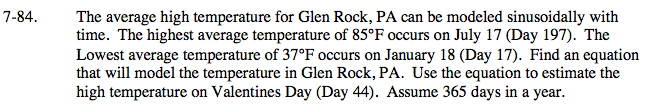### Home > PC > Chapter 7 > Lesson 7.2.4 > Problem7-84

7-84.Plot the given points for the highest temperature and the lowest temperature.

Half of the vertical distance between the low and the high is your amplitude.

Identify the location of the midline. This is the vertical shift.

Double the x-distance between the low and the high point for the period. Then use pb = 2π to find the frequency.

If you use the cosine curve, the horizontal shift would be the x-distance to the first high point. Be careful with your signs.

Then put all of the information in a form such as: f(x) = acos(b(xh)) + k